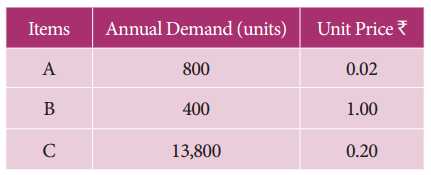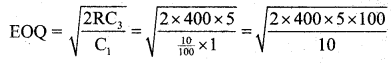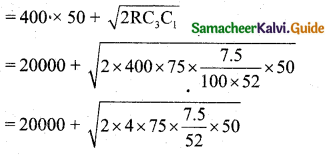Students can download 11th Business Maths Chapter 6 Applications of Differentiation Ex 6.3 Questions and Answers, Notes, Samcheer Kalvi 11th Business Maths Guide Pdf helps you to revise the complete Tamilnadu State Board New Syllabus, helps students complete homework assignments and to score high marks in board exams.

## Tamilnadu Samacheer Kalvi 11th Business Maths Solutions Chapter 6 Applications of Differentiation Ex 6.3

### Samacheer Kalvi 11th Business Maths Applications of Differentiation Ex 6.3 Text Book Back Questions and Answers

Question 1.
The following table gives the annual demand and unit price of 3 itemsOrdering cost is ₹ 5 per order and holding cost is 10% of unit price. Determine the following:
(i) EOQ in units
(ii) Minimum average cost
(iii) EOQ in rupees
(iv) EOQ in years of supply
(v) Number of orders per year
Solution:
Item A:
Demand rate, R = 800
Ordering cost, C3 = ₹ 5
Carrying cost C1 = 10% of unit price
= $$\frac{10}{100}$$ × 0.02

(i) EOQ in units(ii) Minimum Average Cost = C0 = $$\sqrt{2 \mathrm{RC}_{3} \mathrm{C}_{1}}$$
= $$\sqrt{2 \times 800 \times 5 \times \frac{10}{100} \times 0.02}$$
= $$\sqrt{800 \times 0.02}$$
= $$\sqrt{16.00}$$
= ₹ 4(iii) EOQ in rupees = EOQ × Unit price
= 2000 × 0.02
= 2000 × $$\frac{2}{100}$$
= ₹ 40

(iv) $$\frac{\mathrm{EOQ}}{\text { Demand }}=\frac{2000}{800}$$ = 2.5

(v) $$\frac{\text { Demand }}{\mathrm{EOQ}}=\frac{800}{2000}$$ = 0.4

Item B:
Demand rate, R = 400
Ordering cost, C3 = ₹ 5
Carrying cost C1 = 10% of 1.00

(i) EOQ in units= 20 × 10
= 200 units

(ii) Minimum Average Cost = C0 = $$\sqrt{2 \mathrm{RC}_{3} \mathrm{C}_{1}}$$
= $$\sqrt{2 \times 400 \times 5 \times \frac{10}{100} \times 1}$$
= √400
= ₹ 20

(iii) EOQ in rupees = EOQ × unit price
= 200 × 1
= ₹ 200

(iv) $$\frac{\mathrm{EOQ}}{\text { Demand }}=\frac{200}{400}$$ = 0.5

(v) $$\frac{\text { Demand }}{\mathrm{EOQ}}=\frac{400}{200}$$ = 2Item C:
Annual Demand, R = 800
Ordering cost, C3 = ₹ 5
Carrying cost, C1 = 10% of unit price
= $$\frac{10}{100}$$ × 0.20
= $$\frac{2}{100}$$

(i) EOQ in units= 100 × 26.2678
= 100 × 26.27
= 2627

(ii) Minimum Average Cost = C0 = $$\sqrt{2 \mathrm{RC}_{3} \mathrm{C}_{1}}$$
= $$\sqrt{2 \times 13800 \times 5 \times \frac{10}{100} \times 0.2}$$
= $$\sqrt{2 \times 138 \times 5 \times 2}$$
= $$\sqrt{2760}$$
= 52.535
= ₹ 52.54

(iii) EOQ in rupees = 2627 × 0.20 = ₹ 25.40 [∵ Unit price = 0.20]

(iv) $$\frac{\mathrm{EOQ}}{\text { Demand }}=\frac{2627}{13800}$$ = 0.19036 = 0.19

(v) $$\frac{\text { Demand }}{\mathrm{EOQ}}=\frac{13800}{2627}$$ = 5.2531 = 5.25Question 2.
A dealer has to supply his customer with 400 units of a product per week. The dealer gets the product from the manufacturer at a cost of ₹ 50 per unit. The cost of ordering from the manufacturers in ₹ 75 per order. The cost of holding inventory is 7.5 % per year of the product cost. Find
(i) EOQ
(ii) Total optimum cost.
Solution:
Demand = 400 units per week
Annual demand = 400 × 52 per year
Ordering cost per order C3 = 175
Inventory cost C1 = 7.5% per year of the cost
= 7.5% of 50 per year
= $$\frac{7.5}{100}$$ × 50
= $$\frac{7.5 \times 50}{100 \times 52}$$ (per week)
(i) EOQ in unitsEOQ = 912 units(ii) Total optimum cost = Purchasing cost + Minimum annual cost= 20000 + $$\sqrt{\frac{225000}{52}}$$
= 20000 + √4326.92307
= 20000 + √4326.9231
= 20000 + 65.7793
= ₹ 20,065.78 per week